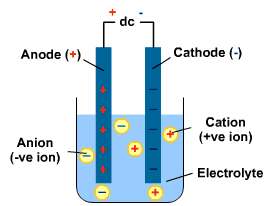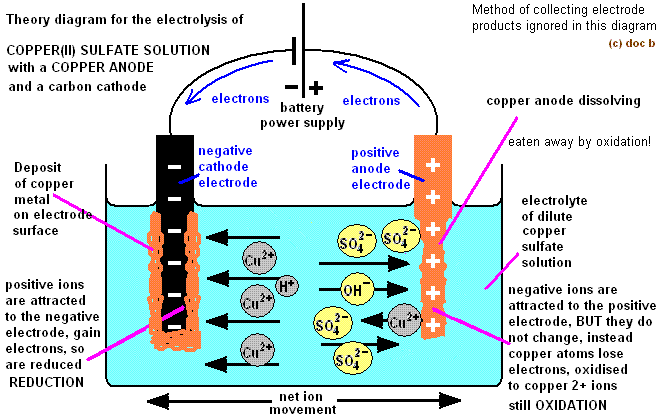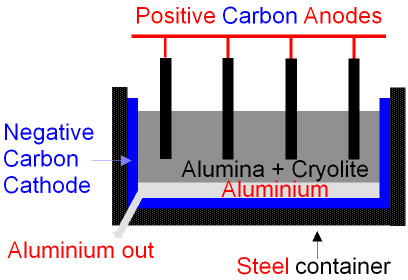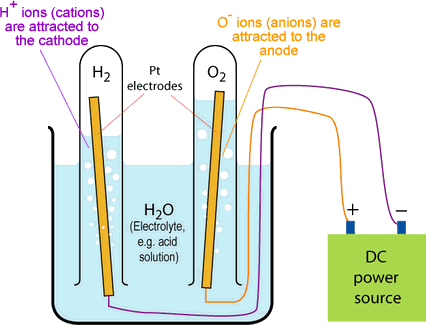# Electrolysis

## Electrolysis

What you need to know:

How electrolysis works and why it is used.

What a redox reaction is and how to use them.

If you put rods into a substance, one rod with a positive change and one with a negative charge. You can create an electric current. When this happens, any charged particles (ions) within the substance are attracted to each side. If the charged particles are free to move, then they move to each side to react with the rods! This decomposes the substance.

Electrolysis: chemical decomposition produced by passing an electric current through a liquid or solution containing ions.

Electrolyte: the liquid containing the ions. It can be a molten ionic substance or a dissolved ionic substance.

Electrode: A solid that conducts electricity and is placed into the electrolyte. A cathode is the negatively charged electrode. The anode is the positively charged electrode.In order for electrolysis to work, the ions need to be free to move.

This can only happen if:

1. There are ions present (for example, in an ionic substance)
2. If the ions are free to move. __This can only happen if the ionic substance is: __

Dissolved in a liquid

Molten (melted).

Redox reactions (HIGHER)

Electrolysis always has reduction reactions and oxidation reactions occuring. Oxidation happens when the negative ions travel to the positive electrode and lose electrons. Reduction occurs when the positive ions move to the negative electrode and gain electrons.

Remember: OILRIG.Half Equations (HIGHER)

Half equations show whats happening at the electrodes.

Here is a half equation for the negative electrode above:You can see that the copper ion has gained two electrons. It took the electrons from the negative electrode.

And here is the half equation for the positive electrode:You can see that the sulfate ion has lost two electrons. It gives the electrons to the positive electrode.

## Electrolysis of Metal Ores

What you need to know:

How electrolysis of metals works and what they are used for.

If a metal is too reactive to be extracted using Carbon, electrolysis can be used.

Aluminium is a metal that needs to be extracted through electrolysis. Bauxite (which is an aluminium ore) can be purified to get aluminium oxide.has a very high melting point so it is dissolved in molten cryolite instead (which brings down the melting point).

Theions are attracted to the negative electrode which is surrounding the bottom of the container. Theions are attracted to the positive anodes. They react with the carbon in the anode to creategas, which bubbles out. This makes the anodes decompose.

The aluminium is now separate from the oxygen so it can be taken away and used.## Electrolysis of Aqueous Solutions

What you need to know:

How electrolysis of aqueous solutions work and what they are used for.

In aqueous solution, there are also OH and H+ ions present, as well as the metal ions. These also get attracted to the anode and cathode. The more reactive an element, the more likely it is to stay in the electrolyte. For example, if the positive metal ion is more reactive than the H+ ion, the metal ion will stay in the electrolyte and the H+ ions will travel to the cathode to collect electrons. They can then bubble out of the chamber as hydrogen gas.

Investigating electrolysisTo investigate electrolysis, you need to set up the equipment as above. The test tubes ensure that any gases are collected.

Turn on the power supply and you should see bubbles coming from each electrode. What these gases are depend on what electrolyte you used.

You can test what gases had been produced by using the common gas tests (see section TestsForCommonGases)